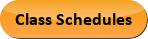Dec 09, 2023
2022-2023 College Catalog
 Select a Catalog 2023-2024 College Catalog 2022-2023 College Catalog [ARCHIVED CATALOG] 2021-2022 College Catalog [ARCHIVED CATALOG] 2020-2021 College Catalog [ARCHIVED CATALOG] 2019-2020 College Catalog [ARCHIVED CATALOG] *** DRAFT 2024-2025 College Catalog DRAFT *** [ARCHIVED CATALOG]
 HELP 2022-2023 College Catalog [ARCHIVED CATALOG] Print-Friendly Page (opens a new window) Add to Portfolio (opens a new window)

# MAT 231 - Calculus II [SUN# MAT 2230]

4 Credits, 4 Contact Hours
4 lecture periods 0 lab periods

Continuation of MAT 220 . Includes techniques and applications of integration, numerical integration, improper integrals, sequences, infinite series, polar coordinates, parametric equations, and other related topics.

Prerequisite(s): Within the last three years: MAT 220  with a grade of C or better.
Gen-Ed: Meets AGEC - MATH; Meets CTE - M&S.Course Learning Outcomes
1. Evaluate indefinite and definite integrals using exact and approximation techniques.
2. Use integrals in various applications.
3. Determine convergence of infinite series.

Performance Objectives:
1. Use definite integrals to calculate areas between curves, volumes of solids, work, arc length, centroids/centers of mass and at least one of the following applications: hydrostatic force, average value of a function, economics, or probability.
2. Calculate indefinite integrals and exact values of definite integrals of algebraic and transcendental functions, including powers and products of trigonometric functions, using substitution, integration by parts, partial fractions, and trigonometric substitutions.
3. Estimate definite integrals using trapezoid, midpoint, and Simpson’s rules.
4. Determine if an improper integral converges or diverges, and if it converges, calculate or estimate its value.
5. Determine if an infinite sequence converges or diverges.
6. Determine if an infinite series converges or diverges using the divergence test, integral test, comparison test, limit comparison test, alternating series test, ratio and root tests.
7. Estimate the error associated with a partial sum approximation of a convergent alternating series
8. Determine if a series converges absolutely or conditionally.
9. Determine radii of convergence and intervals of convergence of power series.
10. Find the power series representation for a given function by integrating or differentiating existing power series.
11. Determine Taylor and Maclaurin series using the definition.
12. Determine slopes and areas of graphs defined in polar coordinates.
13. Determine slopes and arc length of two dimensional curves modeled with parametric equations.

Optional Objectives:

14. Use rationalizing substitutions to calculate indefinite integrals and exact values of definite integrals.

15. Generate the equation of a conic section given a graph, or sketch a graph given an equation.

16. Calculate error bounds for the numerical integration techniques.

17. Generate the binomial series expansion for appropriate functions.

18. Apply integration techniques to solve separable differential equations.

19. Use slope fields and/or Euler’s Method to estimate solutions to differential equations.

Outline:
1. Techniques of Integration
1. Substitution
2. Integration by parts
3. Products and powers of trigonometric functions
4. Partial Fractions
5. Trigonometric substitution
6. Rationalizing substitutions (optional)
7. Numerical integration
1. Trapezoid rule
2. Midpoint rule
3. Simpson’s rule
4. Error bounds (optional)
1. Improper integrals
5. Applications of the Integral
1. Area between curves
2. Volumes of solids
3. Work
4. Centroids or centers of mass
5. Arc length
6. At least 1 of the following:
1. Hydrostatic force
2. Average value of a function
3. Economics
4. Probability
6. Sequences and Series
1. Convergence/divergence of infinite sequences
2. Convergence/divergence of infinite series
1. Divergence test
2. Integral test
3. Comparison test
4. Limit comparison test
5. Alternating series test
6. Ratio test
7. Root test
1. Estimate infinite alternating series
2. Absolute and conditional convergence
3. Power series
1. Radius and interval of convergence
2. Integration and differentiation
3. Taylor and Maclaurin series
4. Binomial series (optional)
1. Parametric Equations and Polar Coordinates
1. Slopes of parametric curves
2. Arc length of parametric curves
3. Slopes of polar curves
4. Areas of polar curves
5. Conic sections (optional)
2. Differential Equations
1. Separable (optional)
2. Slope fields (optional)
3. Euler’s method (optional)

Add to Portfolio (opens a new window)